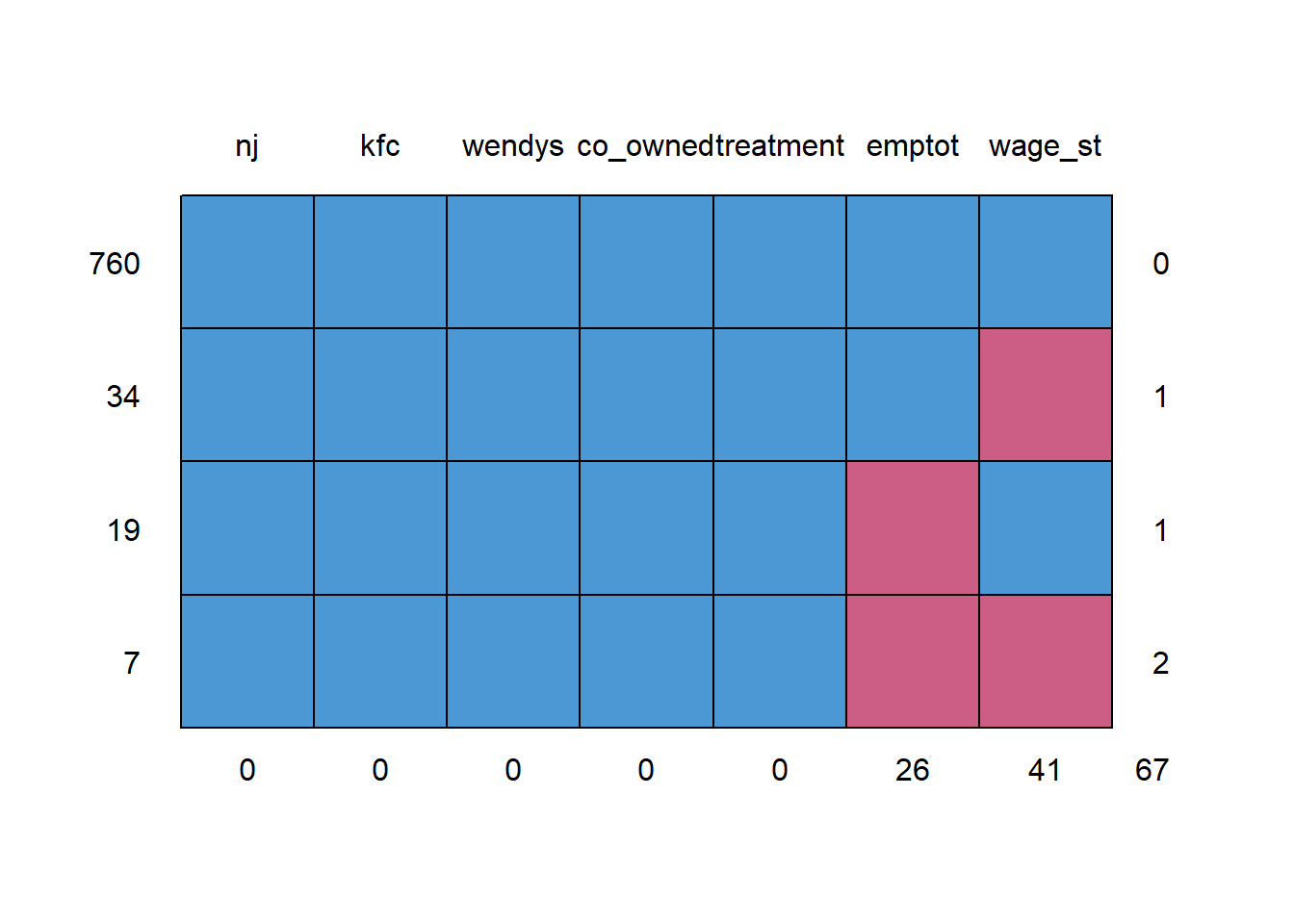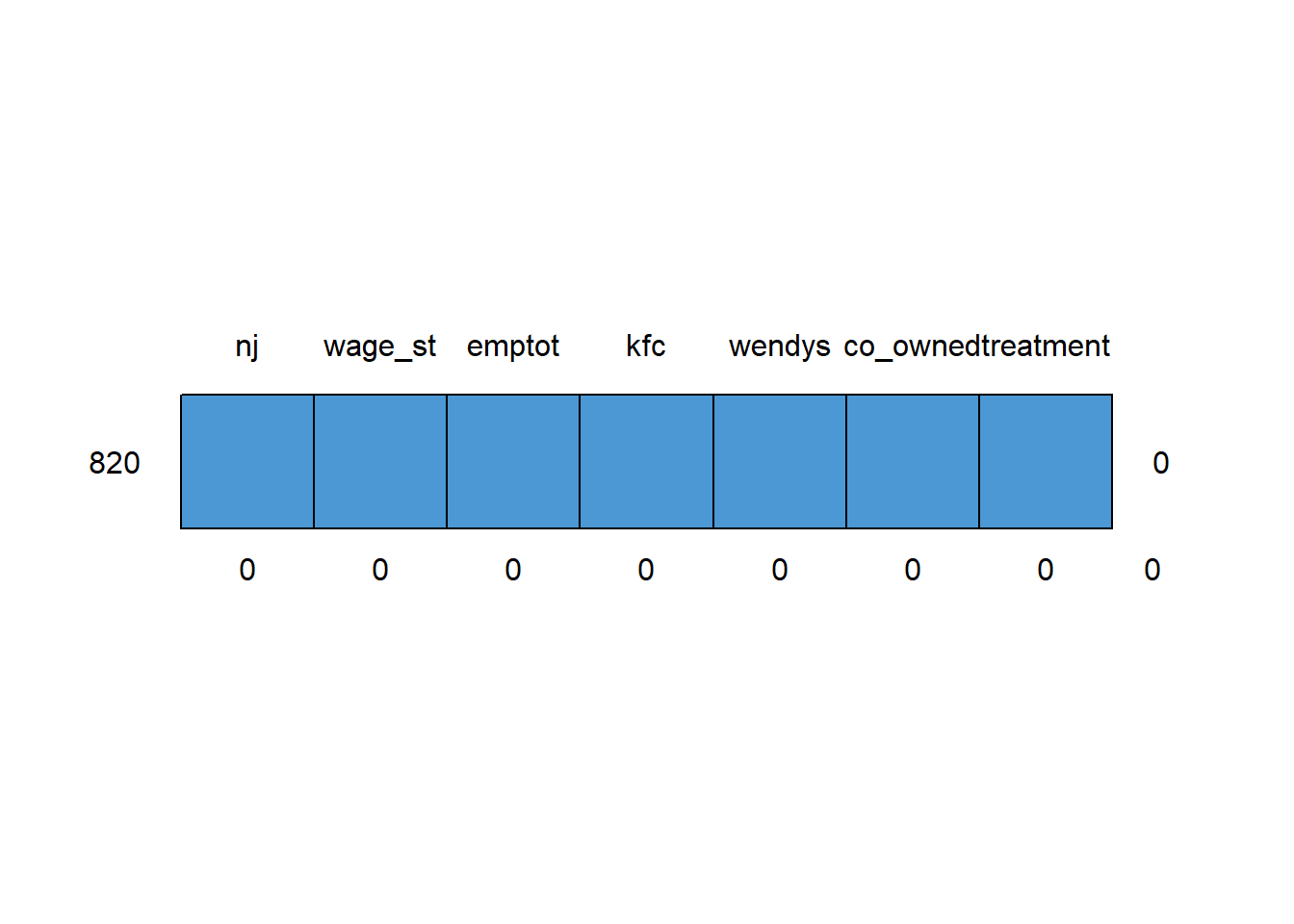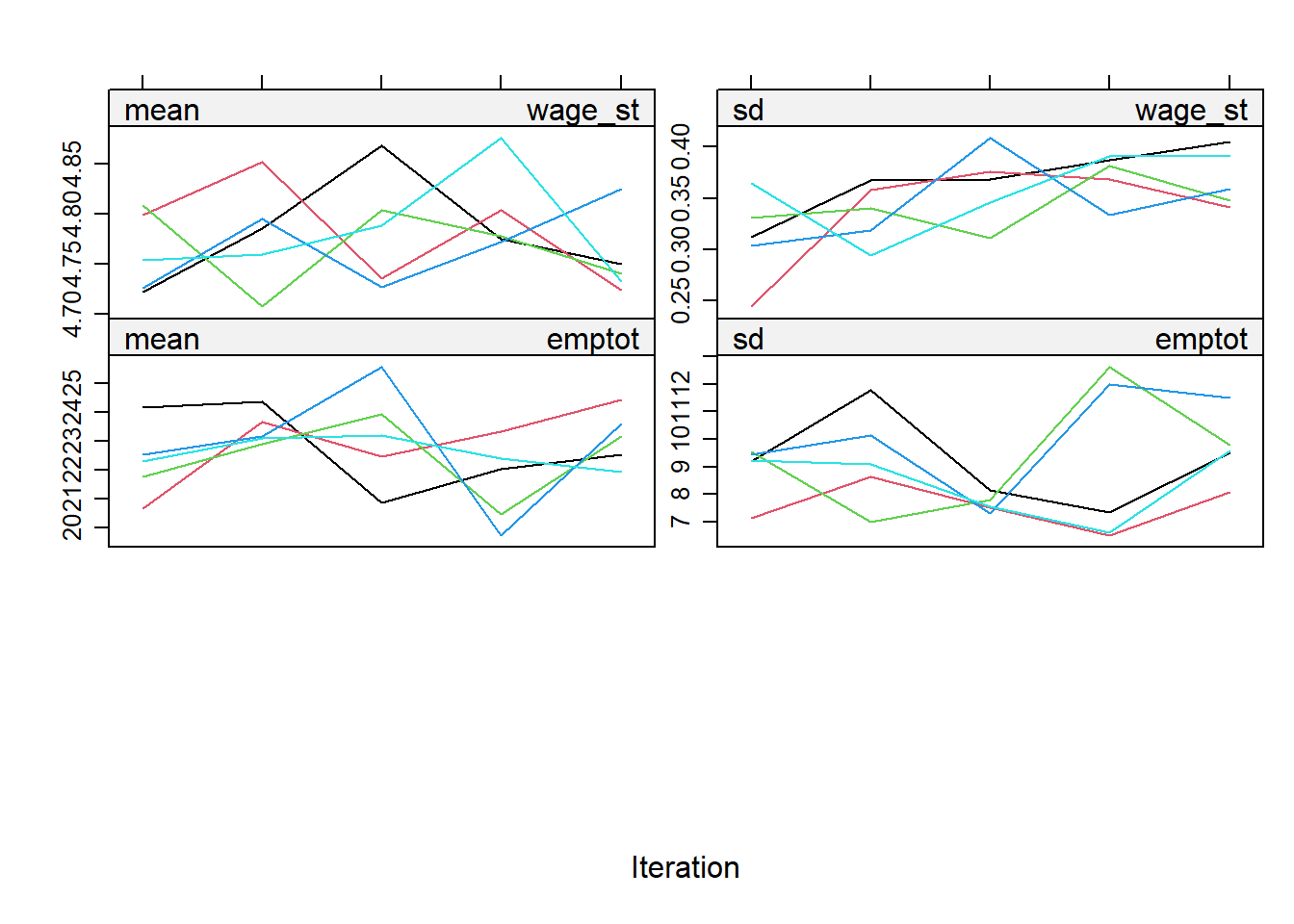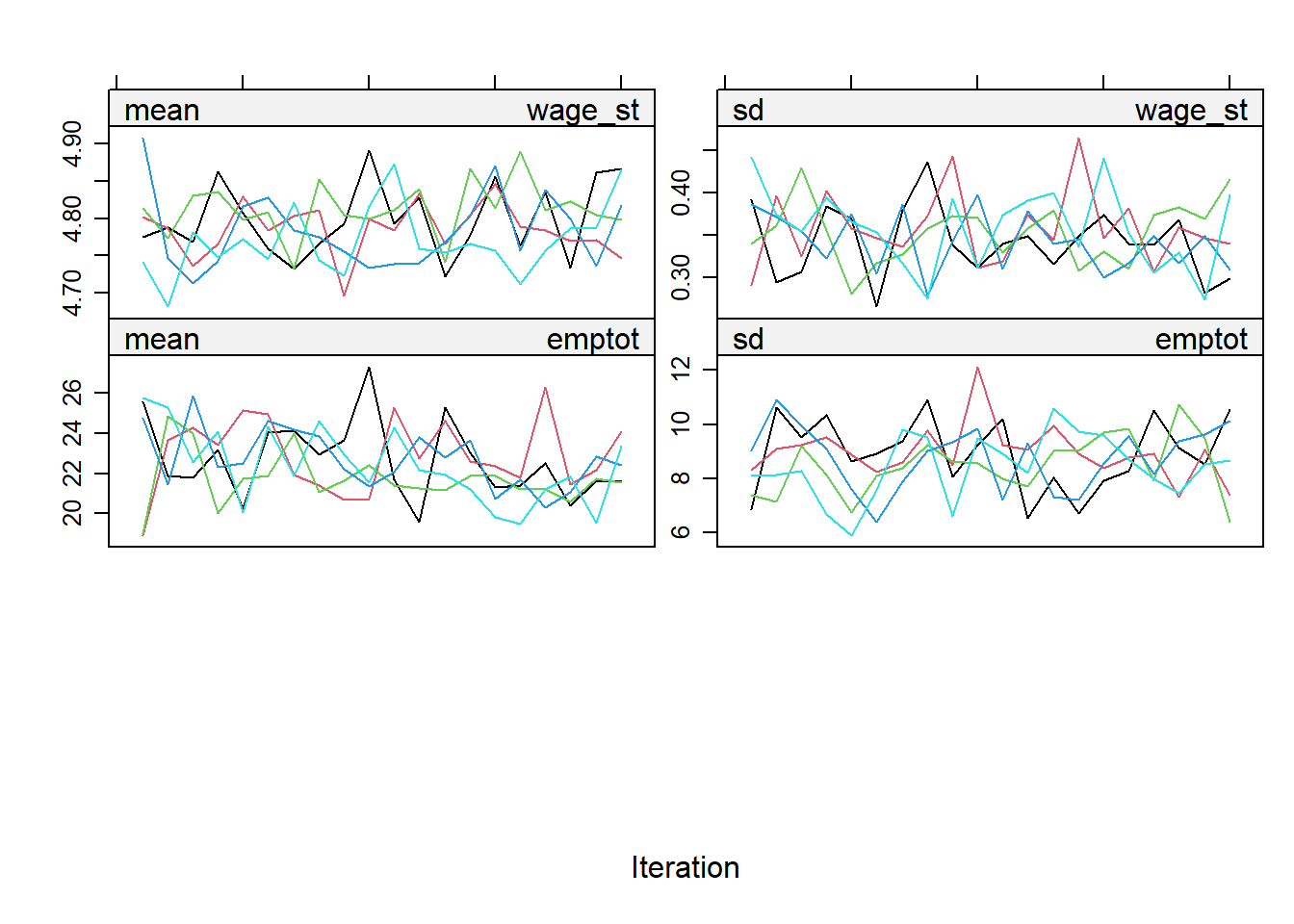# Minimum Wage Analysis with MICE

Author

## Introduction

The aim of this vignette is to replicate and enhance Card and Krueger’s (1994)1 analysis of the effect of an increase in the minimum wage on unemployment by using Multivariate Imputation by Chained Equations (MICE)2.

The aim is to enhance our understanding of multiple imputation and its use for econometric studies. Therefore, this vignette will show how to multiply impute Card and Krueger’s dataset and how to use the imputed data for the econometric analysis. The main objective is to increase your knowledge and understanding on applications of multiple imputation. We use R’s mice package for the analysis3.

## The Economic Analysis

Background: Card and Krueger notorious analysis dealt with analysing the effect that an increase in the minimum wage has on employment levels. We are going to replicate some of their results while using multiple imputation to deal with the missing data.

Experiment: On April 1, 1992, the minimum wage in New Jersey was raised from $4.25 to$5.05. In the neighbouring state of Pennsylvania, however, the minimum wage remained constant at $4.25. Card and Krueger analysed the impact of the minimum wage increase on employment in the fast-food industry, a sector which employs many low-wage workers. Data: The authors collected data on the number of employees in 331 fast-food restaurants in New Jersey and 79 in Pennsylvania. The survey was conducted in February 1992 (before the minimum wage was raised) and in November 1992 (after the minimum wage was raised). The dataset necessary to replicate Card and Krueger’s analysis is available at the author’s website, and here. The dataset is stored in a “wide” format, i.e. there is a single row for each unit (restaurant), and different columns for the outcomes and covariates in different years. The dataset includes the following variables (as well as others which we will not use): Variable name Description nj dummy equal to 1 if the restaurant is located in NJ emptot total number of full-time employed wage\_st average starting wage in the restaurant co\_owned dummy variable equal to 1 if restaurant was co-owned bk dummy variable equal to 1 if restaurant was a Burger King kfc dummy variable equal to 1 if restaurant was a KFC wendys dummy variable equal to 1 if restaurant was Wendys treatment dummy variable equal to 1 if post-treatment For the analysis, the data is presented in a long format. The dataset contains 820 observations on 7 variables: nj, wage_st, emptot, kfc, wendys, co_owned, and treatment. ## Loading wide data min_wage <- read.csv("m_wage.csv", header=TRUE, stringsAsFactors=FALSE) min_wage_feb <- min_wage[,c("nj","wage_st","emptot","kfc", "wendys","co_owned")] min_wage_nov <- min_wage[,c("nj","wage_st2","emptot2","kfc", "wendys","co_owned")] ## Create a treatment period indicator min_wage_feb$treatment <- 0
min_wage_nov$treatment <- 1 ## Make sure the two data.frames have the same column names colnames(min_wage_nov) <- colnames(min_wage_feb) ## Stack the data.frames on top of one another mwl <- rbind(min_wage_feb, min_wage_nov) ## Convert the variables to factors mwl$treatment <- as.factor(mwl$treatment) mwl$nj <- as.factor(mwl$nj) mwl$kfc <- as.factor(mwl$kfc) mwl$wendys <- as.factor(mwl$wendys) mwl$co_owned <- as.factor(mwl\$co_owned)

## Delete the temporary data.frames
rm(min_wage,min_wage_feb,min_wage_nov)

## Missing Data

We load the mice package for multiple imputation. We also set the seed for reproducibility.

library(mice)
set.seed(123)

### Start by exploring the data

Get an overview of the data by the summary() command.

summary(mwl)
 nj         wage_st          emptot      kfc     wendys  co_owned treatment
0:158   Min.   :4.250   Min.   : 0.00   0:660   0:700   0:538    0:410
1:662   1st Qu.:4.500   1st Qu.:14.50   1:160   1:120   1:282    1:410
Median :5.000   Median :20.00
Mean   :4.806   Mean   :21.03
3rd Qu.:5.050   3rd Qu.:25.50
Max.   :6.250   Max.   :85.00
NA's   :41      NA's   :26                                        

Note that there are 41 missing values in wage_st and 26 missing values in emptot.

Using the head() function, we show the first 6 observations.

head(mwl)
  nj wage_st emptot kfc wendys co_owned treatment
1  0      NA  40.50   0      0        0         0
2  0      NA  13.75   1      0        0         0
3  0      NA   8.50   1      0        1         0
4  0     5.0  34.00   0      1        1         0
5  0     5.5  24.00   0      1        1         0
6  0     5.0  20.50   0      1        1         0

The missing values are not randomly distributed across the data set. For example, the missing values in wage_st are concentrated in the post-treatment period, as shown in the first 3 observations. The missing values in emptot are concentrated in the pre-treatment period. This is a common pattern in longitudinal data sets.

### Inspecting the missing data pattern

Check the missingness pattern for the dataset using md.pattern.

md.pattern(mwl)nj kfc wendys co_owned treatment emptot wage_st
760  1   1      1        1         1      1       1  0
34   1   1      1        1         1      1       0  1
19   1   1      1        1         1      0       1  1
7    1   1      1        1         1      0       0  2
0   0      0        0         0     26      41 67

The plot and the table confirm that there are 41 observations with missing values in wage_st and 26 observations with missing values in emptot. Note that there are only 2 observations with both wage_st and emptot missing. The rest of missing values are concentrated in one of the two variables. This means that for most of the observations, we can use the information in one variable to impute the missing values in the other variable. Nonetheless, as described below, MICE can handle the two (or more) missing variables simultaneously.

### Estimating the effect with incomplete data

Effect on employment: In the Difference-in-difference literature4, we estimate the effect of the increase in minimum wage on employment by fitting the model: emptot_{it} = \beta_0 + \beta_1 nj_i + \beta_2 treatment_t + \beta_3 nj_i \times treatment_t + \epsilon_{it}. The effect is then captured by the \beta_3 parameter.

We fit the model with the original dataset. Note that we only need to type nj*treatment in R so that it includes the three regressors (nj,treatment,nj X treatment), they are called the interaction terms.

We fit the model using the with() function. We will cover the use of with() in more detail in the next section. For now, note that with() allows us to fit the linear model using the variables in the desired data frame.

Moreover, we use the lm() function to fit the model. The lm() function is a generic function that can be used to fit a variety of linear models. The summary() function is also generic and can be used to obtain a summary of the fitted model.

fit <- with(mwl, lm(emptot ~ nj*treatment))
summary(fit)

Call:
lm(formula = emptot ~ nj * treatment)

Residuals:
Min      1Q  Median      3Q     Max
-21.166  -6.439  -1.027   4.473  64.561

Coefficients:
Estimate Std. Error t value Pr(>|t|)
(Intercept)      23.331      1.072  21.767   <2e-16 ***
nj1              -2.892      1.194  -2.423   0.0156 *
treatment1       -2.166      1.516  -1.429   0.1535
nj1:treatment1    2.754      1.688   1.631   0.1033
---
Signif. codes:  0 '***' 0.001 '**' 0.01 '*' 0.05 '.' 0.1 ' ' 1

Residual standard error: 9.406 on 790 degrees of freedom
(26 observations deleted due to missingness)
Multiple R-squared:  0.007401,  Adjusted R-squared:  0.003632
F-statistic: 1.964 on 3 and 790 DF,  p-value: 0.118

The estimated effect of the increase in minimum wage on employment is 2.7536058, signalling an increase in total employment in New Jersey after the increase in minimum wage. The standard error is 1.6884091. The effect is not statistically significant at the 5% level. Note that we have used the original dataset with missing values. Hence, we have lost 26 observations.

Effect on wages: We can also estimate the effect on wages by changing the dependent variable in the regression: wage\_st_{it} = \alpha_0 + \alpha_1 nj_i + \alpha_2 treatment_t + \alpha_3 nj_i \times treatment_t + \epsilon_{it}. Analogous to before, the effect is then captured by the \alpha_3 parameter.

fit <- with(mwl, lm(wage_st ~ nj*treatment))
summary(fit)

Call:
lm(formula = wage_st ~ nj * treatment)

Residuals:
Min       1Q   Median       3Q      Max
-0.38013 -0.11213 -0.03085  0.13787  1.63254

Coefficients:
Estimate Std. Error t value Pr(>|t|)
(Intercept)     4.63013    0.03171 146.005   <2e-16 ***
nj1            -0.01800    0.03534  -0.509    0.611
treatment1     -0.01267    0.04563  -0.278    0.781
nj1:treatment1  0.48138    0.05065   9.503   <2e-16 ***
---
Signif. codes:  0 '***' 0.001 '**' 0.01 '*' 0.05 '.' 0.1 ' ' 1

Residual standard error: 0.2765 on 775 degrees of freedom
(41 observations deleted due to missingness)
Multiple R-squared:  0.4073,    Adjusted R-squared:  0.405
F-statistic: 177.5 on 3 and 775 DF,  p-value: < 2.2e-16

The estimated effect is 0.4813823, and the standard error is 0.0506547. The effect is significant at the 1% level, signalling that the policy had the desired effect of increasing wages in New Jersey. Note that we have used the original dataset with missing values. Hence, we have lost 41 observations in this specification.

## Single Imputation Methods

### Basic mean imputation

Imputing the missing data with mean imputation and re-estimating the models.

As a first attempt, we use the mice function, specifying the method = "mean" option. The m option specifies the number of imputations while the maxit option specifies the number of iterations. We use 1 imputation to obtain a single imputed data set. Moreover, we use the print = FALSE option to avoid printing the progress of the algorithm.

imp <- mice(mwl, method = "mean", m = 1, maxit = 1, print = FALSE)

Notice that for the case of imputation with the mean, using mice is extravagant. We could have used the mean() function to compute the mean and the is.na() function to identify the missing values. However, we use mice to illustrate the use of the package.

We can obtain the completed data set using the complete() function that returns a data frame. Here we look at the first 6 observations of the imputed data set.

c1 <- complete(imp, 1)
head(c1)
  nj  wage_st emptot kfc wendys co_owned treatment
1  0 4.805713  40.50   0      0        0         0
2  0 4.805713  13.75   1      0        0         0
3  0 4.805713   8.50   1      0        1         0
4  0 5.000000  34.00   0      1        1         0
5  0 5.500000  24.00   0      1        1         0
6  0 5.000000  20.50   0      1        1         0

Notice that the missing values have been replaced by the mean of the variable. Hence, the 3 first observations have the same value for wage_st, this reduces the variability of the data.

Once we have the imputed data set, we fit the model as before using the with() function.

fit <- with(imp, lm(emptot ~ nj*treatment))
summary(fit)
# A tibble: 4 × 6
term           estimate std.error statistic  p.value  nobs
<chr>             <dbl>     <dbl>     <dbl>    <dbl> <int>
1 (Intercept)       23.3       1.04     22.3  1.09e-86   820
2 nj1               -2.82      1.16     -2.43 1.53e- 2   820
3 treatment1        -2.11      1.47     -1.43 1.52e- 1   820
4 nj1:treatment1     2.68      1.64      1.64 1.02e- 1   820

The estimated effect is 2.6810103, and the standard error is 1.638996.

One of the main drawbacks of using mean imputation in relation to linear regression is that using same value for all missing observations reduces data variability which produces broader confidence intervals. Moreover, mean imputation does not take into account the uncertainty associated with the imputed values. This is reflected in the standard error of the estimated effect, which is smaller than the standard error obtained with the original data.

### Imputation with stochastic regression

One way to add variability to the imputations is to use the method = "norm" option to perform Bayesian normal regression. The idea for the imputation is to fit a Bayesian linear regression with the column with missing data as the dependent variable and using the other variables as regressors. We then use the regression’s estimates to draw a value from the parameters’ posterior distribution to predict the missing value. Furthermore, we add a draw from the estimated distribution of the error term to add variability. The goal is to obtain a different imputed value for each missing observation, which will increase data variability. Nonetheless, note that we have only used 1 imputation.

imp <- mice(mwl, method = "norm", m = 1, maxit = 1, print = FALSE)
c1 <- complete(imp, 1)
head(c1)
  nj  wage_st emptot kfc wendys co_owned treatment
1  0 4.759822  40.50   0      0        0         0
2  0 4.226937  13.75   1      0        0         0
3  0 4.221437   8.50   1      0        1         0
4  0 5.000000  34.00   0      1        1         0
5  0 5.500000  24.00   0      1        1         0
6  0 5.000000  20.50   0      1        1         0

Note that the imputed values for the 3 first observations of wage_st are now different. This increases the variability of the data.

After imputing the missing values, we fit the model once again.

fit <- with(imp, lm(emptot ~ nj*treatment))
summary(fit)
# A tibble: 4 × 6
term           estimate std.error statistic  p.value  nobs
<chr>             <dbl>     <dbl>     <dbl>    <dbl> <int>
1 (Intercept)       23.3       1.06     22.1  6.71e-85   820
2 nj1               -2.79      1.18     -2.37 1.81e- 2   820
3 treatment1        -2.15      1.50     -1.43 1.52e- 1   820
4 nj1:treatment1     2.73      1.66      1.64 1.02e- 1   820

The estimated effect is 2.7291743, and the standard error is 1.6648918. Note that the standard error has increased given the variability in the imputed values. However, we have only used 1 imputation, abstracting from the uncertainty associated with the imputed values. Hence, the standard error misrepresents the uncertainty associated with the fact that we have imputed the missing values.

## Multiple imputation

The motivation behind the use of multiple imputation is to incorporate the uncertainty associated with the imputed values. The idea is to impute the missing values multiple times to obtain multiple imputed data sets. We then fit the model in each imputed data set and combine the results to obtain valid estimates of the parameters of interest.

Suppose that we have m imputed data sets, Y_{miss}^{(1)},\cdots,Y_{miss}^{(m)}, where each is used to obtain an estimate of the effect of interest, \hat{\beta}_i. The goal is to combine the point estimates and the standard errors from the different imputed data sets to obtain confidence valid estimates.

### Posterior mean

Assuming that the estimate is unbiased on the complete data, \bar{\beta}_m defined as \bar{\beta}_m = \frac{1}{m}\sum_{i=1}^m \hat{\beta}_i, is an unbiased estimator for the true effect \beta.

Hence, we can pool the different estimates to obtain \bar{\beta}_m as our point estimate for \beta. All that is left to do is to obtain the standard error of \bar{\beta}_m.

### Posterior variance: Three sources of variation

By the Law of Total Variance, the posterior variance of P(\beta|Y_{obs}) is Var(\beta|Y_{obs}) = E\left[Var(\beta|Y_{obs},Y_{miss})\right|Y_{obs}] + Var\left[E(\beta|Y_{obs},Y_{miss})\right|Y_{obs}].

The first term is called the within-imputation variance, and the second term is the between-imputation variance.

The within-imputation variance is the expectation of the variance of the estimate if we use a complete dataset conditional on the current set of imputed values. It is estimated by: \bar{U}_m = \frac{1}{m}\sum_{i=1}^m \hat{U}_i, where \hat{U}_i is the within-imputation variance of \hat{\beta}_i.

The between-imputation variance is estimated by: \bar{B}_m = \frac{1}{m-1}\sum_{i=1}^m \left(\hat{\beta}_i - \bar{\beta}_m\right)\left(\hat{\beta}_i - \bar{\beta}_m\right)', which can be interpreted as the variance of the point estimates across the different imputed data sets.

We further need to incorporate the variance due to the fact that we are estimating \bar{\beta}_m for finite m. This is called the simulation variance and it can be estimated by \bar{B}_m/m.

In summary, the total variance T_m of \bar{\beta} is decomposed as T_m = \bar{U}_m + \bar{B}_m + \frac{\bar{B}_m}{m}, where:

• \bar{U}_m, the variance given that we are taking a sample rather than observing the entire population;
• \bar{B}_m, the variance given that there are missing values in the sample;
• \bar{B}_m/m, the variance given that we use a finite amount of imputations.

Note that we must use T_m to obtain the standard error of \bar{\beta}_m and compute confidence intervals with valid coverage. Hence, we use multiple imputation to account for all the sources of variation.

The mechanism to combine the estimates and obtain valid tests and confidence intervals are called Rubin’s rules. We will describe how to use Rubin’s rules in mice below.

### Multiple imputations using mice

Obtaining multiple imputations is straightforward in mice by modifying the m option. Using the complete() function shows the imputed data. Here, we examine the second imputed data set using md.pattern().

imp <- mice(mwl, method = "norm", m = 5, maxit=5, print = FALSE)
c2 <- complete(imp, 2)
md.pattern(c2)
 /\     /\
{  ---'  }
{  O   O  }
==>  V <==  No need for mice. This data set is completely observed.
\  \|/  /
-----'nj wage_st emptot kfc wendys co_owned treatment
820  1       1      1   1      1        1         1 0
0       0      0   0      0        0         0 0

As expected, there are not more missing values in the imputed data set. The missing values have been replaced by imputed values. Analogously, we can examine the additional imputed data sets using complete().

### How many imputations?

As shown above, the number of imputations directly influences the total variance of the estimates. As we have done before, we can modify the number of imputations by using the m option. The question then arises as to how many imputations are needed.

The answer is that we need enough imputations to obtain stable estimates. The number of imputations should be large enough to obtain stable estimates, typically m\geq 5. The default in mice is m= 5. More imputations are needed when the proportion of missing data is high. Nowadays, computational power is not a limitation so we can use m=20 or m=100.

Additionally, some authors suggest to repeat the analysis with different seeds. If there are large differences between experiments, this could indicate that the observed data contain little information about the effects of interest.

### Chained equations

The mice() function uses a Markov Chain Monte Carlo (MCMC) type of algorithm to obtain the imputations. The algorithm is called chained equations because it imputes the missing values one variable at a time connecting the imputations in a chain. The algorithm is as follows:

1. Start with a simple imputation method to generate a complete data set (Y_{obs},Y_{imp}^{(1)}).
2. For each variable in the dataset Y_{imp}^{(i)}, impute the missing values using the other variables in the complete dataset. This produces new imputed values Y_{imp}^{(i+1)}.
3. Repeat steps 1-2 several times, up to a maxit number of times, to obtain stable estimates in one complete dataset.
4. Repeat steps 1-3 m times to obtain multiple imputed data sets.

Given the MCMC nature of the method, we should always inspect the convergence of the algorithm. We can look at the trace lines generated by the algorithm to study convergence.

plot(imp)There is one line for each imputed dataset through the iterations. The plots here show 5 iterations, the default value for maxit. The idea is that the algorithm has converged when the trace lines are stable and do not show any trend. Here, the standard deviation for the imputed values of wage_st is perhaps not stable as it seems to be increasing.

One way to deal with the lack of convergence is to increase the number of iterations. We can do this by changing the value passed to the maxit option. Here, we increase the number of iterations to 20 to see if the trace lines become stable.

imp <- mice(mwl, method = "norm", m = 5, maxit = 20, print = FALSE)
plot(imp)Note that the algorithm has converged for all variables. The trace lines are stable and do not show any trend.

### Diagnostics

Another important consideration regarding the imputed values is that they should be valid. That is, the imputed values should be plausible given the observed data and physical/logical restrictions.

We can use the stripplot() function to compare the imputed values to the observed values. The function plots the imputed values, in red, together with the observed values, in blue, for each imputed data set. The first column shows only the observed data for comparison purposes. The idea is that the imputed values should be valid in the sense that they are similar to the observed ones.

stripplot(imp, pch = 20, cex = 2)